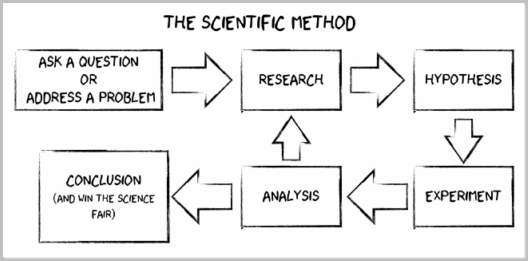# The five steps of the scientific method

The scientific method is a five-step process used in scientific investigation. Sometimes the steps are combined or added to, but the five are the basic structure for any endeavor to answer a question objectively.## Step 1: Ask a question or identify a problem

A scientist learns about the universe by observing. Scientific investigations are carried out to answer a question based on observations – who, what, when, where, how. Sometimes that question is seeking a solution to a problem. The first step in the scientific process is for the investigator to frame the problem to be solved or ask a question that lacks an answer in a way that is falsifiable. Falsifiability is the concept that an observation can show that an answer is contradicted (i.e. you cannot prove that something is correct only that it has exceptions or is not correct).

## Step 2: Background research

Sometimes combined with step three, the investigator starts addressing the question by gathering information about the question that will aid in designing experiments and making observations. The process involves background research and becoming familiar with what is already known about the question and its potential answer, including any wrong answers that were previously identified.

## Step 3: Form a hypothesis

Based on an educated guess, the investigator attempts to answer the question they asked in step one. Based on current knowledge, previous solutions and current practice, a hypothesis is proposed that can be tested as true or false, yes or no. The potential answer to the question is stated in a way that allows for a single answer in order to determine if the hypothesis is correct.

## Step 4: Experiment and observe

The hypothesis is tested by experimentation and observation. Experiments are designed to have one variable, with the other factors involved remaining constant. By changing a single variable at a time, a fair test is conducted that provides objective results about the effect of that specific variable in relation to the controlled conditions.

The investigator records all observations and measurements for the experiments for later analysis. The experiments are repeated multiple times to ensure the results are credible and to account for human error and contamination. Multiple conditions and variables are often tested as well.

## Step 5: Draw a conclusion

After completing the experiments, the results are analyzed to determine whether the hypothesis was true or false. If the hypothesis is true, then the question is answered. If the hypothesis is false, what was learned is integrated and a new hypothesis formed, including subsequent experiments and observation.

## Scientific investigation

This process is repeated as often as needed to reach a conclusion based on observation and experimentation. For many types of scientific investigations, the conclusion leads to more questions and more hypotheses. A scientist is never done searching for answers.

For examples of the use of the scientific method, see the teacher resources at University of Rochester and Science Buddies.